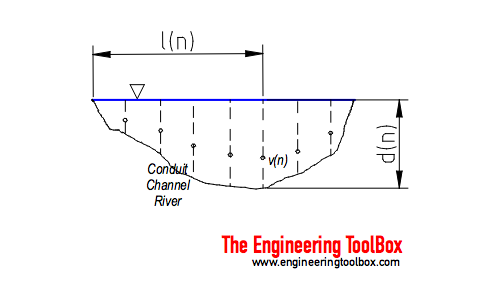Engineering ToolBox - Resources, Tools and Basic Information for Engineering and Design of Technical Applications!

# Velocity-Area Flowmetering

## Flow rate or discharge in an open conduit, channel or river can be calculated with the velocity-area principle.

The velocity-area principle is based on velocity measurements in a open flow like a conduit, channel or river.Velocities and depths across the stream are measured as indicated in the figure above. A partial discharge in a section of the stream can be calculated as

qn = vn an              (1)

where

qn = flow rate or discharge in section n    (m3/s, ft3/s)

vn = measured velocity in section n   (m/s, ft/s)

an = area of section n   (m2, ft2

One simple way to express the section area is

an = dn (ln+1 - ln-1) / 2                              (2)

The total flow in the stream can be summarized to

Q = Σ1n vn an                         (3)

where

Q = summarized flow rate or discharge in the conduit   (m3/s, ft3/s)

The accuracy of estimate depends on the profile of the conduit and the number of measurements. For conduits with regular shapes like rectangular channels a limited number of measurements are required. For irregular shapes - like natural rivers or similar - higher accuracy requires more measurements both horizontal and vertical.

### Example - Computing Flow Rate in a Channel

From a conduit we have three measurements:

Computing Flow Rate in a Channel
Measured ValuesCalculated Values
nv
(m/s)
d
(m)
l
(m)
a
(m2)
q
(m3/s)
0 0 0 0
1 3 1 2 2 6
2 4 1.5 4 3 12
3 3 0.9 6 1.8 5.4
4 0 0 8
Summarized 23.4

The section areas can be calculated like

a1 = (1 m) ((4 m) - (0 m)) / 2

= 2 m2

a2 = (1.5 m) ((6 m) - (2 m)) / 2

= 3 m2

a3 = (0.9 m) ((8 m) - (4 m)) / 2

= 1.8 m2

The flow rates can be calculated as

q1 = (3 m/s) (2 m2)

= 6 m3/s

q2 = (4 m/s) (3 m2)

= 12 m3/s

q3 = (3 m/s) (1.8 m2)

= 5.4 m3/s

The total flow can be summarized as

Q = (6 m3/s) + (12 m3/s) + (5.4 m3/s)

= 23.4 m3/s

Note - there are alternative ways to calculate the section flow rates:

### Simple Average Method

Using the simple average of two successive vertical depths, their mean velocity, and the distance between them can be expressed as

qn to n+1  = [(vn + vn+1) / 2] [(dn + dn+1 ) / 2] (ln+1 - ln)                  (4)

### Midsection Method

With the midsection method, the depth and mean velocity are measured for each number of verticals along the cross section. The depth at a vertical is multiplied by the width, which extends halfway to the preceding vertical and halfway to the following vertical, to develop a cross-sectional area. The section flow rate can be expressed as

qn = vn [((ln - ln-1) + (ln+1 - ln)) / 2] dn                 (5)

## Related Topics

• ### Flow Measurement

Flow metering principles - Orifice, Venturi, Flow Nozzles, Pitot Tubes, Target, Variable Area, Positive Displacement, Turbine, Vortex, Electromagnetic, Ultrasonic Doppler, Ultrasonic Time-of-travel, Mass Coriolis, Mass Thermal, Weir V-notch, Flume Parshall and Sluice Gate flow meters and more.

## Related Documents

• ### Comparing Flowmeters

A limited comparison of flowmeter principles - regarding service, rangeability, pressure loss, typical accuracy, upstream pipe diameters, viscosity and relative costs.
• ### Flowmeter - Accuracy

Introduction to accuracy in flow measurement devices.
• ### Flowmeters - Turndown Ratios

Turndown ratio (Rangeability) can be used to compare flow measurement devices like orifices, venturi meters etc.
• ### Fluid Flowmeters - Comparing Types

An introduction to the different types of fluid flowmeters - Orifices, Venturies, Nozzles, Rotameters, Pitot Tubes, Calorimetrics, Turbine, Vortex, Electromagnetic, Doppler, Ultrasonic, Thermal, Coriolis.
• ### Hydrostatic Pressure vs. Depth

Depth and hydrostatic pressure.
• ### Open Channel Weirs - Volume Flow Measurements

Weirs can be used to measure flow rates in open channels and rivers - common for water supply and sewage plants.
• ### Orifice, Nozzle and Venturi Flow Rate Meters

The orifice, nozzle and venturi flow rate meters makes the use of the Bernoulli Equation to calculate fluid flow rate using pressure difference through obstructions in the flow.
• ### Sluice Gate - Volume Flow Measurements

Sluice gates can be used to control and measure volume flow rates in open channels and rivers, mainly in connection to hydro power plants.
• ### U-Tube Differential Pressure Manometers

Inclined and vertical u-tube manometers used to measure differential pressure in flow meters like pitot tubes, orifices and nozzles.

• ### Volume Flow - Online Unit Converter

Convert between volume flow units like gpm, liter/sec, cfm, m3/h.
• ### Water - Volume Flow Measurement

A manual providing guidance in selecting, managing, inspecting, and maintaining water measurement devices - describes standard methods and devices commonly used to measure irrigation water.

## Engineering ToolBox - SketchUp Extension - Online 3D modeling!

Add standard and customized parametric components - like flange beams, lumbers, piping, stairs and more - to your Sketchup model with the Engineering ToolBox - SketchUp Extension - enabled for use with older versions of the amazing SketchUp Make and the newer "up to date" SketchUp Pro . Add the Engineering ToolBox extension to your SketchUp Make/Pro from the Extension Warehouse !

We don't collect information from our users. More about

## Citation

• The Engineering ToolBox (2017). Velocity-Area Flowmetering. [online] Available at: https://www.engineeringtoolbox.com/velocity-area-computing-flow-principle-d_1974.html [Accessed Day Month Year].

Modify the access date according your visit.

10.2.10